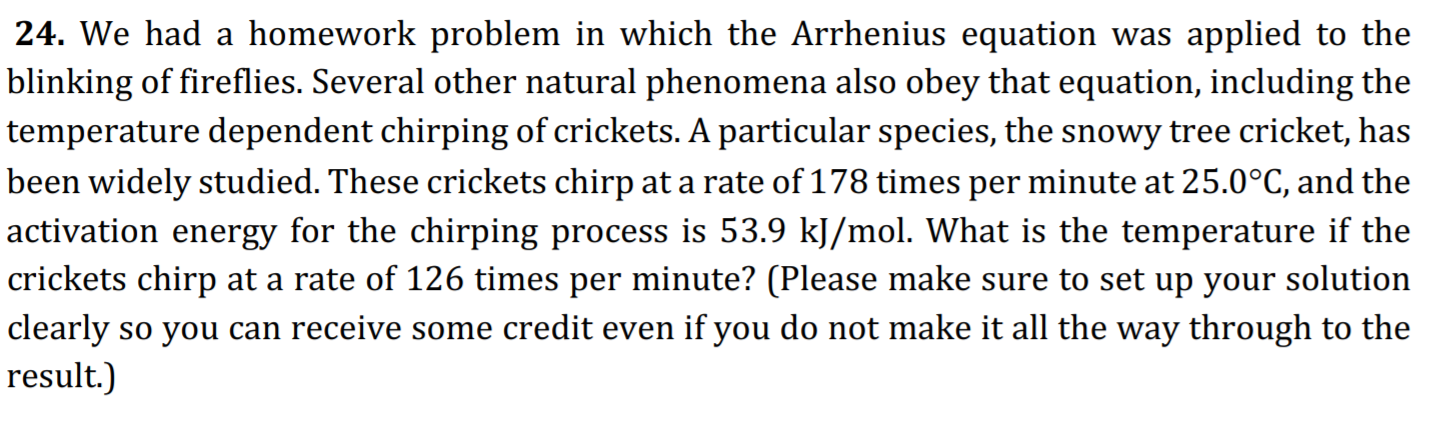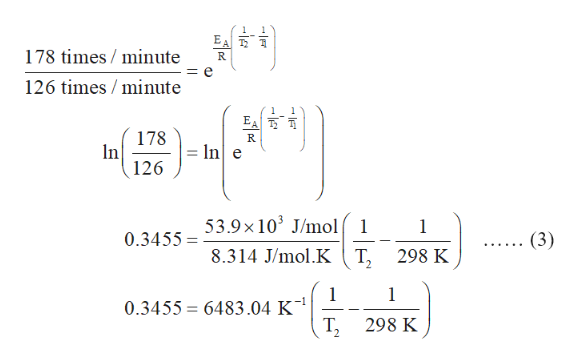# 24. We had a homework problem in which the Arrhenius equation was applied to theblinking of fireflies. Several other natural phenomena also obey that equation, including thetemperature dependent chirping of crickets. A particular species, the snowy tree cricket, hasbeen widely studied. These crickets chirp at a rate of 178 times per minute at 25.0°C, and theactivation energy for the chirping process is 53.9 kJ/mol. What is the temperature if the|crickets chirp at a rate of 126 times per minute? (Please make sure to set up your solutionclearly so you can receive some credit even if you do not make it all the way through to theresult.)

Question
101 viewshelp_outlineImage Transcriptionclose24. We had a homework problem in which the Arrhenius equation was applied to the blinking of fireflies. Several other natural phenomena also obey that equation, including the temperature dependent chirping of crickets. A particular species, the snowy tree cricket, has been widely studied. These crickets chirp at a rate of 178 times per minute at 25.0°C, and the activation energy for the chirping process is 53.9 kJ/mol. What is the temperature if the |crickets chirp at a rate of 126 times per minute? (Please make sure to set up your solution clearly so you can receive some credit even if you do not make it all the way through to the result.) fullscreen
check_circle

Step 1

The relation between degree Celsius (C)and Kelvin (K) is expressed below.

T(K) = 273 + T(C)

Use the above relation and Convert 25 degrees Celsius into Kelvin.

T (K) = 273 + 25C

T(K) = 298 K

The relation between kilojoule/mole (kJ/mol) and joule/mole (J/mol) is expressed below.

1 kJ/mol = 1 ×103 J/mol

Convert 53.9 kJ/mol into J/mol.

53.9 kJ/mol = 53.9 ×103 J/mol

Step 2

The ratio of two rate constants by using Arrhenius equation as expressed in equation (1).

Step 3

Substitute the values of rate constants, 53.9 ×103 J/mol for EA, 298 K fo...help_outlineImage TranscriptioncloseEA 178 times / minute 126 times / minute 178 In 126 R = In] e 53.9 × 10' J/mol 1 (3) 0.3455 = 8.314 J/mol.K ( T, 298 K 0.3455 = 6483.04 K¯' т, 298 K fullscreen

### Want to see the full answer?

See Solution

#### Want to see this answer and more?

Solutions are written by subject experts who are available 24/7. Questions are typically answered within 1 hour.*

See Solution
*Response times may vary by subject and question.
Tagged in

### Chemistry